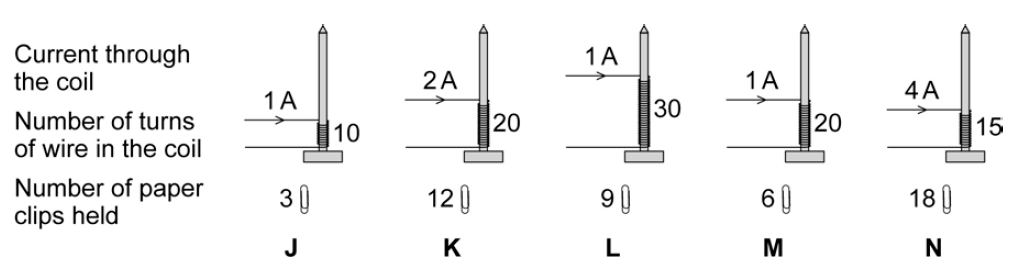# Electromagnetism – Question 1

The diagram shows the equipment used by a student to investigate the strength of five different electromagnets.The stronger the electromagnet, the more paper clips it will hold.

(a) Why is it important that the paper clips used in the investigation are all the same size?(1 mark)

(b) The five electromagnets, J, K, L, M and N, used by the student are shown below.

Each electromagnet was made by wrapping lengths of insulated wire around identical iron nails.The student wants to find out how the strength of an electromagnet depends on the number of turns of wire in the coil.

Which electromagnets should the student compare in order to do this?(1 marks)

The student concluded:

‘The strength of an electromagnet is always directly proportional to the number of turns on the coil.’

(c) (i) Explain how the data from the investigation supports the student’s conclusion.(2 marks)

(c) (ii) The student makes one more electromagnet by winding 100 turns onto a nail.

Before testing the electromagnet, the student predicted the number of paper clips that the electromagnet would hold when the current is 1 amp.

How many paper clips should the student predict that the electromagnet would hold?Number of paper clips = ..................

(2 marks)

(c) (iii) When the student tested the electromagnet it held 20 paper clips. This is not what the student predicted.

Explain what the student should do when new data does not seem to support the prediction that was made.(3 marks)

How did you do? Click to show the mark schemeFrom Q2 P3 AQA Specimen Paper (Higher)